### Practice Set 7.1 Mensuration Class 10th Mathematics Part 2 MHB Solution

Practice Set 7.1
1. Find the volume of a cone if the radius of its base is 1.5 cm and its perpendicular…
2. Find the volume of a sphere of diameter 6 cm.
3. Find the total surface area of a cylinder if the radius of its base is 5 cm and height…
4. Find the surface area of a sphere of radius 7 cm.
5. The dimensions of a cuboid are 44 cm, 21 cm, 12 cm. It is melted and a cone of height…
6. Observe the measures of pots in figure 7.8 and 7.9. How many jugs of water can the…
7. A cylinder and a cone have equal bases. The height of the cylinder is 3 cm and the area…
8. In figure 7.11, a toy made from a hemisphere, a cylinder and a cone is shown. Find the…
9. In the figure 7.12, a cylindrical wrapper of flat tablets is shown. The radius of a…
10. Figure 7.13 shows a toy. Its lower part is a hemisphere and the upper part is a cone.…
11. Find the surface area and the volume of a beach ball shown in the figure.…
12. As shown in the figure, a cylindrical glass contains water. A metal sphere of diameter…
###### Practice Set 7.1
Question 1.

Find the volume of a cone if the radius of its base is 1.5 cm and its perpendicular height is 5 cm.

Radius of base of cone, r = 1.5cm

Perpendicular height of cone, H = 5cm

As we know that,

Volume of the cone,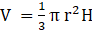On substituting the given values,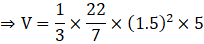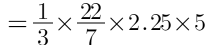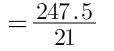⇒ V = 11.79 cm3

∴ Volume of the cone is 11.79 cm3

Question 2.

Find the volume of a sphere of diameter 6 cm.

Diameter of the sphere, d = 6 cm

⇒ Radius of sphere, r = 3 cm

As we know, the volume of sphere,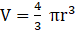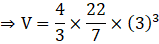⇒ V = 113.04 cm3

∴ Volume of sphere is 113.04 cm3

Question 3.

Find the total surface area of a cylinder if the radius of its base is 5 cm and height is 40 cm.

Radius of base of cylinder, r = 5 cm

Height of cylinder, H = 40 cm

As we know,

Total surface area of cylinder, A = 2πr (r + h)

On substituting the values, we get,

A = 2× (3.14)× 5× (5 + 40)

⇒ A = 1413 sq.cm

∴ Total surface area of square is 1413 sq. cm

Question 4.

Find the surface area of a sphere of radius 7 cm.

Radius of sphere, r = 7cm

As we know, the surface area of sphere, A = 4πr2

On substituting the values, we get,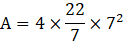⇒ A = 616 sq. cm

∴ Surface area of sphere is 616 sq. cm

Question 5.

The dimensions of a cuboid are 44 cm, 21 cm, 12 cm. It is melted and a cone of height 24 cm is made. Find the radius of its base.

Since the volume of cuboid = length× breadth× height

⇒ Volume of cuboid = Product of the given three dimensions

⇒ Volume of cuboid = 44× 21× 12

Height of cone, H = 24 cm

Let Radius of cone be r

Volume of cone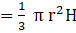As the cone is melted to form a cone,

⇒ Volume of cone = volume of cuboid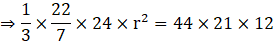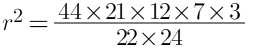r2 = 21× 7 × 3

r2 = 21× 21

⇒ r = 21 cm

∴ The radius of the cone is 21 cm

Question 6.

Observe the measures of pots in figure 7.8 and 7.9. How many jugs of water can the cylindrical pot hold?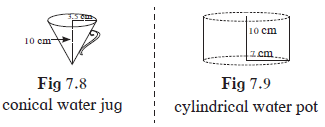Height of water jug, HJ = 10cm

Radius of water jug, RJ = 3.5 cm

Volume of conical jug,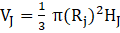Let the number of jugs be n.

Height of cylindrical pot, HP = 10 cm

Radius of pot, RP = 7 cm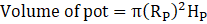Since the water is transferred from pot to ‘n’ number of jugs,

⇒ Volume of pot = n × Volume of jug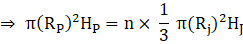On substituting the given values,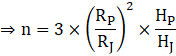⇒ n = 3× 22× 1

⇒ n = 12 cm

∴ The cylindrical pot can hold 12 jugs of water.

Question 7.

A cylinder and a cone have equal bases. The height of the cylinder is 3 cm and the area of its base is 100 cm2.The cone is placed upon the cylinder. Volume of the solid figure so formed is 500 cm3. Find the total height of the figure.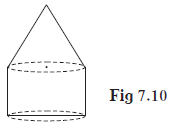Let the radius of base be r.

Let the height of cone = H

Height of cylinder, h = 3cm

Area of base, A = 100 sq. cm

As we know the area of circle is πr2

⇒ πr2 = 100 ….. (1)

Volume of complete solid figure,V = Volume of cone + volume of cylinder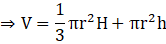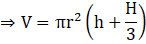It is given that volume of solid figure, V = 500 cubic cm

On substituting the value of V and πr2 from eq (1), we get,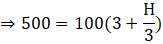⇒ H = 6 cm

Total height of figure = h + H = 3 + 6 = 9 cm

∴ total height is 9 cm

Question 8.

In figure 7.11, a toy made from a hemisphere, a cylinder and a cone is shown. Find the total area of the toy.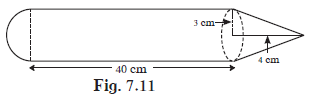Radius of circular region, r = 3cm

Height of Cylinder, H = 40cm

Height of cone, h = 4cm

As we know,

Surface area of hemisphere, AH = 2πr2

Surface area of cylinder, Acy = 2πrH

Surface area of cone is,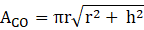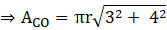ACO = 5πr

Total area = AH + ACY + ACO

On substituting the values, we get,

⇒ Total area = πr(2r + 2H + 5)

⇒ Total area = π× 3× (6 + 80 + 5)

⇒ Total area = 273π

∴ total area of the figure is 273π sq. cm

Question 9.

In the figure 7.12, a cylindrical wrapper of flat tablets is shown. The radius of a tablet is 7 mm and its thickness is 5 mm. How many such tablets are wrapped in the wrapper?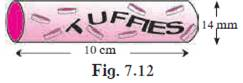Since there are only flat tablets which are stacked onto each other inside the cylindrical wrapper, so the radius of the wrapper does not matter.

Height of wrapper, H = 10cm = 100mm

Thickness of the tablet, h = 5mm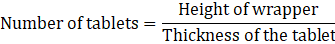⇒ Number of the tablets = 20

∴ There are 20 tablets inside the cylindrical wrapper.

Question 10.

Figure 7.13 shows a toy. Its lower part is a hemisphere and the upper part is a cone. Find the volume and the surface area of the toy from the measures shown in the figure.(π= 3.14)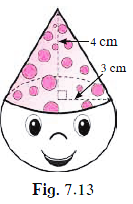Radius of the circular base, r = 3cm

Height of cone, h = 4cm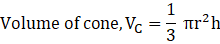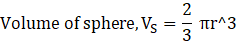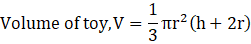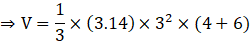⇒ V =3.14×3 × 10

⇒ V = 94.20 cubic cm

Now,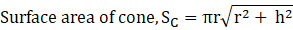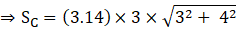⇒ SC = 3.14 × 3 × 5

⇒ SC = 47.1 sq.cm

Surface area of sphere, SS = 2πr2

⇒ SS = 2× (3.14) × 32

⇒ SS = 56.52 sq.cm

Surface area of toy = 47.1 + 56.52 = 103.52 sq.cm

∴ Volume and surface area of toy is 94.20 cm3 and 103.52 cm2 respectively

Question 11.

Find the surface area and the volume of a beach ball shown in the figure.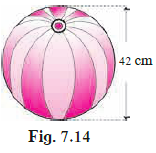Diameter of spherical ball, d = 42 cm

Radius of ball, r =21 cm

As we know that, Surface area of sphere, A = 4πr2

⇒ A = 4× (3.14) × (21)2

⇒ A = 5538.96 sq.cm

Also,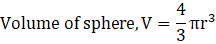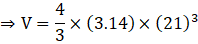⇒ V = 38772.72 cm3

∴ The surface area and volume of the spherical ball is 5538.96 cm2 and 38772.72 cm3 respectively

Question 12.

As shown in the figure, a cylindrical glass contains water. A metal sphere of diameter 2 cm is immersed in it. Find the volume of the water.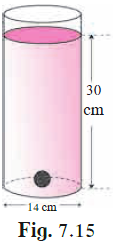Diameter of glass, D = 14 cm

⇒ Radius of glass, r = 7cm

Height of water level, H = 30 cm

We know that, volume of cylinder, V = πR2H

⇒ V = π × (7)2× 30

⇒ V = 1470π cubic cm

Diameter pof metal sphere, d = 2 cm

Radius of metal sphere, r = 1cm

As we know that,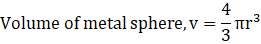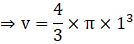⇒ v = 1.33π cubic cm

Volume of water = Volume of glass – Volume of metal sphere

⇒ Volume of water = 1470π – 1.33π

∴ volume of the water is 1468.67π cm3

## PDF FILE TO YOUR EMAIL IMMEDIATELY PURCHASE NOTES & PAPER SOLUTION. @ Rs. 50/- each (GST extra)

SUBJECTS

HINDI ENTIRE PAPER SOLUTION

MARATHI PAPER SOLUTION
SSC MATHS I PAPER SOLUTION
SSC MATHS II PAPER SOLUTION
SSC SCIENCE I PAPER SOLUTION
SSC SCIENCE II PAPER SOLUTION
SSC ENGLISH PAPER SOLUTION
SSC & HSC ENGLISH WRITING SKILL
HSC ACCOUNTS NOTES
HSC OCM NOTES
HSC ECONOMICS NOTES
HSC SECRETARIAL PRACTICE NOTES

2019 Board Paper Solution

HSC ENGLISH SET A 2019 21st February, 2019

HSC ENGLISH SET B 2019 21st February, 2019

HSC ENGLISH SET C 2019 21st February, 2019

HSC ENGLISH SET D 2019 21st February, 2019

SECRETARIAL PRACTICE (S.P) 2019 25th February, 2019

HSC XII PHYSICS 2019 25th February, 2019

CHEMISTRY XII HSC SOLUTION 27th, February, 2019

OCM PAPER SOLUTION 2019 27th, February, 2019

HSC MATHS PAPER SOLUTION COMMERCE, 2nd March, 2019

HSC MATHS PAPER SOLUTION SCIENCE 2nd, March, 2019

SSC ENGLISH STD 10 5TH MARCH, 2019.

HSC XII ACCOUNTS 2019 6th March, 2019

HSC XII BIOLOGY 2019 6TH March, 2019

HSC XII ECONOMICS 9Th March 2019

SSC Maths I March 2019 Solution 10th Standard11th, March, 2019

SSC MATHS II MARCH 2019 SOLUTION 10TH STD.13th March, 2019

SSC SCIENCE I MARCH 2019 SOLUTION 10TH STD. 15th March, 2019.

SSC SCIENCE II MARCH 2019 SOLUTION 10TH STD. 18th March, 2019.

SSC SOCIAL SCIENCE I MARCH 2019 SOLUTION20th March, 2019

SSC SOCIAL SCIENCE II MARCH 2019 SOLUTION, 22nd March, 2019

XII CBSE - BOARD - MARCH - 2019 ENGLISH - QP + SOLUTIONS, 2nd March, 2019

HSC Maharashtra Board Papers 2020

(Std 12th English Medium)

HSC ECONOMICS MARCH 2020

HSC OCM MARCH 2020

HSC ACCOUNTS MARCH 2020

HSC S.P. MARCH 2020

HSC ENGLISH MARCH 2020

HSC HINDI MARCH 2020

HSC MARATHI MARCH 2020

HSC MATHS MARCH 2020

SSC Maharashtra Board Papers 2020

(Std 10th English Medium)

English MARCH 2020

HindI MARCH 2020

Hindi (Composite) MARCH 2020

Marathi MARCH 2020

Mathematics (Paper 1) MARCH 2020

Mathematics (Paper 2) MARCH 2020

Sanskrit MARCH 2020

Important-formula

THANKS